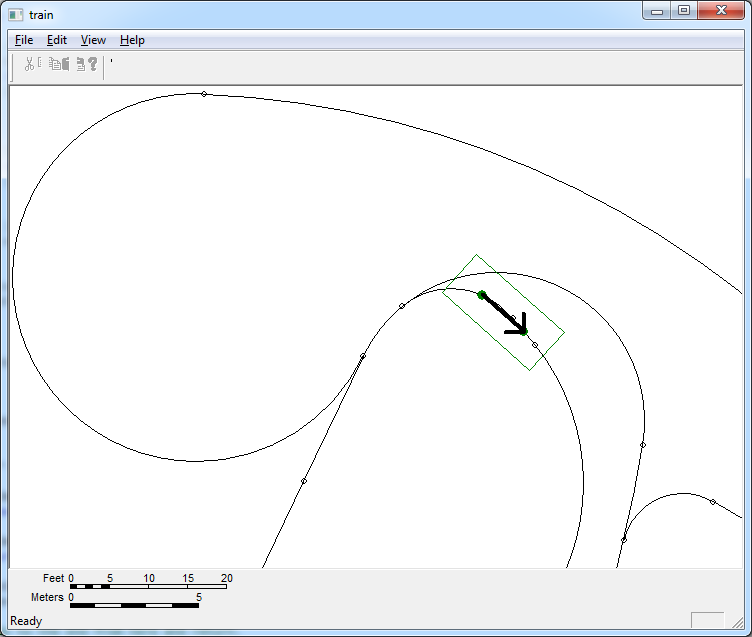I have a working sample of the code I mentioned in an older blog post. I have a self-propelled train car that moves along any unending track layout. When the front wheels of the train car passes over a turnout, a random choice is made by this train and that choice is kept until the train has passed the turnout. Here’s a picture:The green rectangle with two green dots and an arrow is the train car. The arrow points to the logical front of the car.

Some of the code is a little complex, like the code used to calculate the location of the front bogie on one of the track segments. Here is some of that code, which shows my simplistic and less-than-optimal method of doing the needed work:

```
bool CTrainImplementation::FindLinearLocation( const CTrackNetworkLocation &TrackLocation, int Direction, double LinearDistance, CTrackNetworkLocation &NewLocation )
{
/*
* Determine if the new point in on the current segement and use the
* distance to the end of the segment to determine which point to use
* if there are two possible locations. If the point is not on the current
* segment, step through each segment in the specified direction and find
* one that is the proper distance from us. As soon as both ends of the
* next segment are beyond the linear distance, stop and report that there
* is no new location. THIS MIGHT NOT BE OPTIMAL IN SOME SITUATIONS OF
* UNUSUAL TRACK CONFIGURATIONS.
*/

if( TrackLocation.Segment() == 0 )
return false;

CCircle Circle( TrackLocation.Point(), LinearDistance );

bool bDoDirectionTest = true;

CSegment *pSegment = TrackLocation.Segment();

while( pSegment != 0 )
{
CArcLine ArcLine = *pSegment;

CFPoint Point0;
CFPoint Point1;
bool bIntersects = Intersects( ArcLine, Circle, Point0, Point1 );

if( bIntersects )
{
if( Point0 == Point1 )
{
if( bDoDirectionTest )
{
/*
* We are on the same segment as the starting point
* so the direction needs to be tested. If the one
* point is in the wrong direction then the point is
* ignored.
*/

CArcLine TestArcLine = ArcLine;
CArcLine TestArcLine0 = ArcLine;
TestArcLine.SetDistance( TrackLocation.SegmentDistance() );
TestArcLine0 = Point0;
// Check to see if test arcline is longer or shorter than before.
int TempDirection = TestArcLine0.GetAngularDistance() > TestArcLine.GetAngularDistance() ? 1 : 0;
if( TempDirection != Direction )
bIntersects = false;
}
}
else
{
ArcLine.SetDistance( TrackLocation.SegmentDistance() + ( Direction == 0 ? -LinearDistance : +LinearDistance ) );
double Distance0 = GetDistance( ArcLine, Point0 );
double Distance1 = GetDistance( ArcLine, Point1 );
if( Distance1 < Distance0 )
Point0 = Point1;
}
}

if( bIntersects )
{
ArcLine.m_End = Point0;
double NewDistance = ArcLine.GetDistance();
NewLocation.Set( pSegment, ArcLine.GetDistance(), TrackLocation.Orientation() );
break;
}

CWayPoint *pWayPoint = pSegment->GetWayPoint( Direction );
CSegment *pNextSegment = GetNextRouteSegment( pSegment, Direction );

CWayPoint *pNewSegmentWayPoint = pNextSegment->GetWayPoint( Direction );
if( pNewSegmentWayPoint == pWayPoint )
Direction = Direction == 0 ? 1 : 0;

pSegment = pNextSegment;

bDoDirectionTest = false;
}

return pSegment != 0;
}
```

You can see that I over-use some geometry objects and also use them like compass and straight edge. I will work to find better ways to calculate the results of some of these operations without creating geometric objects for everything. Some of the most expensive operations are things like setting the distance of an arcline which does sine and cosine computations. Distance calculations are also expensive.

I won’t explain the classes used here. It is just the computational burden that I’m trying to convey. As another example of this, the code to move a point along the track network that is needed for train movement is also fairly complex but uses a bit less geometry.

I’ll try to embed an animated version of this when I get a chance. By then, I’ll also have multi-car trains.